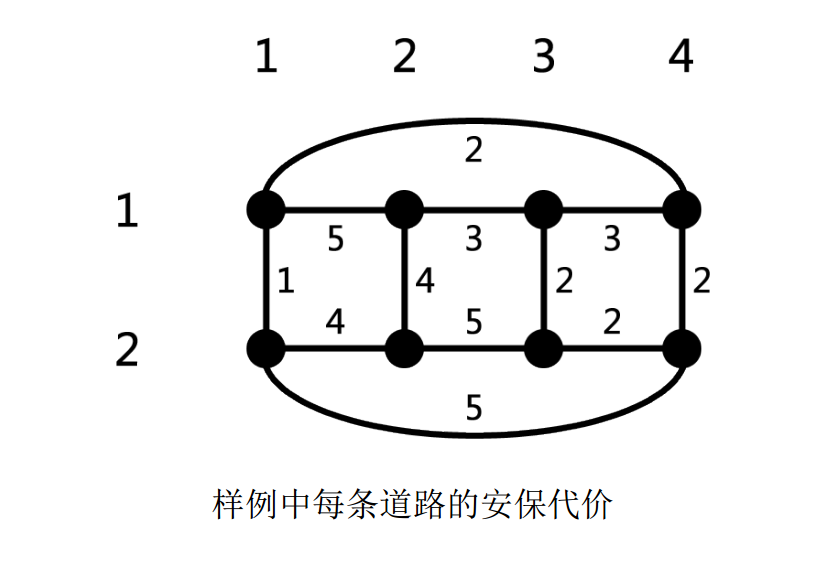# #3112. 「SDOI2019」世界地图

#### 输入格式

unsigned int SA, SB, SC;int lim;
int getweight() {
SA ^= SA << 16;
SA ^= SA >> 5;
SA ^= SA << 1;
unsigned int t = SA;
SA = SB;
SB = SC;
SC^ = t ^ SA;
return SC % lim + 1;
}
void gen() {
scanf("%d%d%u%u%u%d", &n, &m, &SA, &SB, &SC, &lim);
int i, j, w;
for(i = 1; i <= n; i++)
for(j = 1; j <= m; j++) {
w = getweight();
if (j < m) {
addedge(i, j, i, j + 1, w);
} else {
}
}
for(i = 1; i < n; i++)
for(j = 1; j <= m; j++) {
w = getweight();
addedge(i, j, i + 1, j, w);
}
}


#### 样例输入

2 4 1 2 3 5
3
2 2
2 3
3 3


#### 样例输出

9
5
13


#### 样例说明